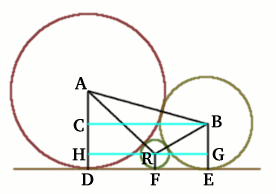#### You may also like### Kissing

Two perpendicular lines are tangential to two identical circles that touch. What is the largest circle that can be placed in between the two lines and the two circles and how would you construct it?### Logosquares

Ten squares form regular rings either with adjacent or opposite vertices touching. Calculate the inner and outer radii of the rings that surround the squares.### Just Touching

Three semi-circles have a common diameter, each touches the other two and two lie inside the biggest one. What is the radius of the circle that touches all three semi-circles?

# Baby Circle

##### Age 16 to 18 Challenge Level:Rosalind of Madras College sent in this solution, well done Rosalind.

All three circles touch each other and have a common tangent $DFE$. The radius of the baby circle is $r$ and the radii of the other circles are $BE = 1$ unit and $AD = 2$ units. So $AC = 1$ unit, $AB = 3$ units, $AH = 2 - r$, $AR = 2 +r$, $BR = 1 + r$ and $BG = 1 - r$. Using Pythagoras Theorem: \eqalign{ \; RG &=& \sqrt{(1+r)^2 - (1-r)^2} = \sqrt{4r} = 2\sqrt{r} \\ \; HR &=& \sqrt{(2+r)^2 - (2-r)^2} = \sqrt{8r} = 2\sqrt{2r} \\ \; CB &=& 2\sqrt{2} = DE \\ RG + HR = CB &\Rightarrow& 2\sqrt{r} + 2\sqrt{2r} = 2\sqrt{2}.} This gives $\sqrt{r}(1+\sqrt{2}) = \sqrt{2}$ and hence by squaring \eqalign{ r &=& \frac{2}{3+2\sqrt{2}} \\ \; &=& \frac{2(3 - 2\sqrt{2})}{(3+2\sqrt{2})(3-2\sqrt{2})} \\ \; &=& \frac{6 - 4\sqrt{2}}{9 - 4\times2} \\ \; &=& 6 - 4\sqrt{2}} So the radius of the baby circle is $6 - 4\sqrt{2}$.